Courses

# Quant Mock Test - 5

## 15 Questions MCQ Test Mock Test Series for CLAT 2020 | Quant Mock Test - 5

Description
This mock test of Quant Mock Test - 5 for CLAT helps you for every CLAT entrance exam. This contains 15 Multiple Choice Questions for CLAT Quant Mock Test - 5 (mcq) to study with solutions a complete question bank. The solved questions answers in this Quant Mock Test - 5 quiz give you a good mix of easy questions and tough questions. CLAT students definitely take this Quant Mock Test - 5 exercise for a better result in the exam. You can find other Quant Mock Test - 5 extra questions, long questions & short questions for CLAT on EduRev as well by searching above.
QUESTION: 1

### Directions: Study the following carefully and answer the questions that follow: Percentage of employees in 7 departments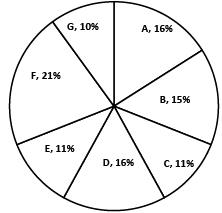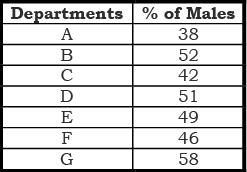Q. If the number of male employees in department A is 12160, what is the number of employees in department D?

Solution:

38/100 × x = 12160
So total employees in department A, x = 32000
Since % of employees in departments A and D is same
So employees in department D = 32000

QUESTION: 2

### Directions: Study the following carefully and answer the questions that follow: Percentage of employees in 7 departmentsQ. In the number of employees in department D is 32000, then find the number of male employees in department B.

Solution:

16/100 × x = 32000
So total employees in company, x = 200,000
So male employees in B = 52/100 × 15/100 × 200000 = 15600

QUESTION: 3

### Directions: Study the following carefully and answer the questions that follow: Percentage of employees in 7 departmentsQ. Find the ratio of male employees in department F to that in department C.

Solution:

46 × 21 : 42 × 11

QUESTION: 4

Directions: Study the following carefully and answer the questions that follow:
Percentage of employees in 7 departmentsQ. If the number of male employees in department A is 12160, then find the number of employees in department E.

Solution:

38/100 × 16/100 × x = 12160
So total employees in company, x = 200,000
So employees in department E = 11/100 × 200,000 = 22,000

QUESTION: 5

Directions: Study the following carefully and answer the questions that follow:
Percentage of employees in 7 departmentsQ. The number of employees in department B is 30, 000. If next year, the number of employees in departments B and G increase by 10% each, then find the number of male employees in department G next year.

Solution:

15/100 × x = 30,000
So total employees in company in present year, x = 200,000
So total employees in G in present year = 10/100 × 200,000 = 20,000
So total employees in G in next year = 110/100 × 20,000 = 22,000
So male employees = 58/100 × 22000 = 12760

QUESTION: 6

Directions: Study the following piechart and table carefully and answer the questions given below:
Percentage of people in a city working in the night shift from various industries
(Total number of people 50250)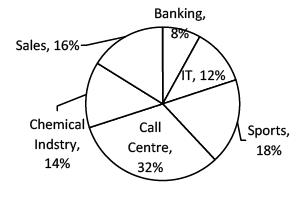Percentage of females from various industries in the night shift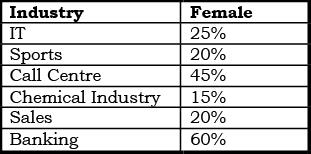Q. What is the approximate average number of people working in the night shift from IT, Banking and Sales together?

Solution:

Required Average =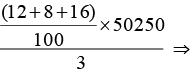6030

QUESTION: 7

Directions: Study the following piechart and table carefully and answer the questions given below:
Percentage of people in a city working in the night shift from various industries
(Total number of people 50250)Percentage of females from various industries in the night shiftQ. What is the approximate number of males working in the night shift from Sports and chemical Industry together?

Solution:

Total no. of males in sports and chemical = 80% of 18% of 50250 + 85% of 14% of 50250
= 7236 + 5979.75
≈ 13216

QUESTION: 8

Directions: Study the following piechart and table carefully and answer the questions given below:
Percentage of people in a city working in the night shift from various industries
(Total number of people 50250)Percentage of females from various industries in the night shiftQ. The number women working in the night shift from the Call Centre is what per cent of the total number of people working in the night shift from Sports?

Solution:

Number of women working in call centre =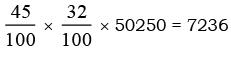Number of person working in sports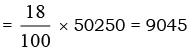Required percentage =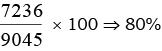QUESTION: 9

Directions: Study the following piechart and table carefully and answer the questions given below:
Percentage of people in a city working in the night shift from various industries
(Total number of people 50250)Percentage of females from various industries in the night shiftQ. What is the difference between the number of males working in the night shift from Sales and the number of females working in the night shift form Banking?

Solution:

Required difference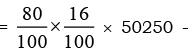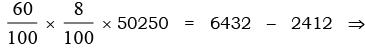4020

QUESTION: 10

Directions: Study the following piechart and table carefully and answer the questions given below:
Percentage of people in a city working in the night shift from various industries
(Total number of people 50250)Percentage of females from various industries in the night shiftQ. The number of males working in the night shift from IT is what per cent of the total number of males working in the night shift from Sports?

Solution:

Required percentage =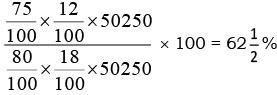QUESTION: 11

Directions: Study the following graph carefully and answer the questions given below: Preferences of people for different professions over the years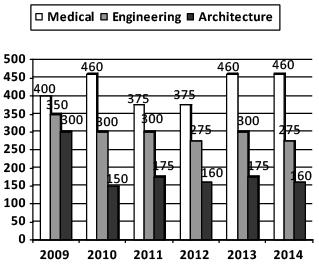Q. From 2009 to 2014, the total number of people who preferred Engineering as their profession, was approximately how many million?

Solution:

350 + 300 + 300 + 275 + 300 + 275 = 1800

QUESTION: 12

Directions: Study the following graph carefully and answer the questions given below: Preferences of people for different professions over the yearsQ. The number of people preferring Engineering in 2014 was how many million fewer than the number of people preferring Engineering in 2009?

Solution:

350 – 275 = 75

QUESTION: 13

Directions: Study the following graph carefully and answer the questions given below: Preferences of people for different professions over the yearsQ. In 2011, the no. of people who preferred Medical as their profession is approximately what percent of the number of people who preferred Medical, Engineering and Architecture together as their profession in the same year?

Solution: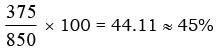QUESTION: 14

Directions: Study the following graph carefully and answer the questions given below: Preferences of people for different professions over the yearsQ. What is the ratio of the number of people who preferred Medical to that of those who preferred Engineering in the year of 2012?

Solution:

The correct option is A.

Number of people preferred medical in 2012 = 375

Number of people preferred engineering in 2012 = 275

Ratio = 375/275

= 15/11

QUESTION: 15

Directions: Study the following graph carefully and answer the questions given below: Preferences of people for different professions over the yearsQ. In the year 2013, the Architecture course fee reduces by 50%. Due to the reduction in fees, 50% of the people who had preferred Engineering as their profession, now prefer Architecture. How many million of people prefer Architecture now?

Solution:

50% of 300 = 150
150 + 175 = 325